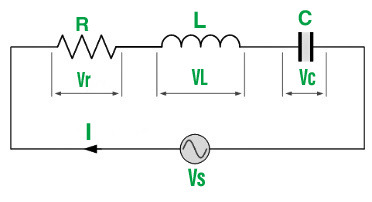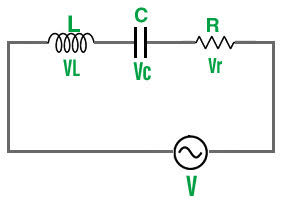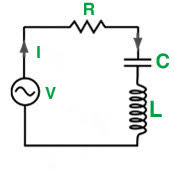GFG App
Open AppBrowser
Continue

In contrast to direct current (DC), which travels solely in one direction, Alternating Current (AC) is an electric current that occasionally reverses direction and alters its magnitude constantly over time. Alternating current is the type of electricity that is delivered to companies and homes, and it is the type of electricity that is used by consumers when they plug in kitchen appliances, televisions, fans, and electric lamps to a wall outlet. A flashlight’s battery cell is a frequent source of DC power. When modifying current or voltage, the abbreviations AC and DC are frequently used to signify merely alternating and direct.

In most electric power circuits, the most common waveform of alternating current is a sine wave, whose positive half-period correlates to the positive current direction and vice versa. The current may not truly reverse direction (as for the labeled pulsating waveform). Different waveforms, such as triangle waves or square waves, are employed in various applications, such as guitar amplifiers. Alternating current also includes audio and radio signals transmitted by electrical lines. Information like sound (audio) or images (video) is occasionally transmitted via modulation of an AC carrier signal in these forms of alternating current. The frequency of these currents is usually higher than that of power transmission currents.

### Series LCR Circuit

An LCR circuit is made up of three components: an inductor (L), a capacitor (C), and a resistor (R). A tuned or resonant circuit is another name for it. A series LCR circuit is made up of these devices that are connected in series. As a result, the resistor, capacitor, and inductor will all have the same amount of current flowing through them.A voltage VS is applied across the LCR series circuit in the above circuit, which depicts a simple LCR series circuit.
Impedance is the amount of resistance a circuit provides to current flow. It’s the effective resistance to alternating current flow in an electric circuit made up of numerous electric components. It is caused by the interaction of ohmic resistance, capacitive reactance, and inductive reactance. If R denotes resistance, XL denotes inductive reactance, XC denotes capacitive reactance, then Z denotes impedance.

Z=√ R2+(XC−XL)2

### Derivation for AC Voltage applied across Series LCR CircuitAn inductor (L), capacitor (C), and resistor (R) are linked in series in the electrical circuit, which is powered by an AC voltage supply. The alternating voltage V is supplied by the voltage source, where

V=Vm sin(ωt)

where,

• Vm is the amplitude of the applied voltage, and
• ω is the frequency of the applied voltage.

If q is the charge on the capacitor and I is the current flowing in the circuit at any moment t, the voltage equation for the circuit can be written as follows:

Net EMF across the circuit: V (source voltage) = Voltage drop across resistor + Voltage drop across capacitor + Self-induced Faraday’s emf in the inductor

V=L(di/dt) + IR + q/C

The inductor’s self-inductance is denoted by L.

Substituting alternating voltage for the expression,

Vm sin(ωt) = L(di/dt) + IR + q/C          …..(1)

Let us use the analytical method to determine the instantaneous current I or its matching phase to the applied alternating voltage V. We know that current is equal to the rate at which electric charge flows per unit of time, i.e.,

I=dq/dt

Differentiating both sides with respect to time, we get:

dI/dt=d2q/dt2

The voltage equation in terms of q is obtained by substituting the above value into equation (1):

Vm sin(ωt) = L(d2q/dt2) + (dq/dt)R + q/C             ……(2)

The equation for a forced or damped harmonic oscillator is similar to this equation.

q = qm sin(ωt+θ)

Differentiating both sides with respect to time,

dq/dt = qm ωcos(ωt+θ)

d2q/dt2=–qm ω2sin(ωt+θ)

Substituting these values in equation (2),

Vm sin(ωt) = qm ω [Rcos(ωt+θ) + (XC–XL)sin(ωt+θ)]                 …..(3)

Here,

• Capacitive reactance: XC=1/ωC
• Inductive reactance: XL=ωL
• Impedance: Z= √R2+(XC−XL)2

Substituting the above values in equation (3), so we get:

Vm sin(ωt)=qm ωZ[R/Z cos(ωt+θ) + (XC–XL)/Zsin(ωt+θ)]                         ……(4)

Consider,

R/Z = cos∅

(XC–XL)/Z = sin∅

Dividing the two equations:

(XC–XL)/R=tan∅

∅=tan–1((XC–XL)/R)

Substituting the above values in equation (4):

Vm sin(ωt)=qm ωZ[cos(ωt+θ–∅)]

Comparing the LHS and RHS of this equation, we get

Vm=qm ωZ=Im Z

The current in the LCR circuit,

I=dq/dt

or,

I = qm ωcos(ωt+θ)

I = Im cos(ωt+θ)                        [where, qm ω=Im]

Since, θ–∅= – π/2

θ= – π/2 + ∅

We get,

I = Im cos(ωt–π/2+∅)

I = Im sin(ωt+∅)

Here, Im=Vm/Z = Vm / √R2+(XC–XL)2 and ∅=tan–1(XC–XL/R)

• Thus, for  θ=0 , As a result, the applied voltage and instantaneous current are in phase
• For θ=90 , The applied voltage is out of phase with the instantaneous current.

### Resonance of LCR Circuit

If the output of a circuit reaches its maximum at a specific frequency, it is said to be in resonance. The resonance phenomenon is connected with systems that have a tendency to oscillate at a specific frequency known as the natural frequency of the system. The amplitude of oscillation is observed to be considered when an energy source drives such a system at a frequency close to the natural frequency.We discovered that the amplitudes of voltage, frequency, and current are related to each other in the following series of LCR circuits:

Im = Vm/Z = Vm / √R2+(XC–XL)2

where,

• XC=1/ωC and
• XL=ωL

Im=Vm/Z=Vm / √R2+(1/ωC−ωL)2

When the circuit’s impedance is low, the current flowing through it is at its maximum. To accomplish so, we change the frequency value till we have XC=XL at a given frequency of ω0 and the impedance,

Z=√ R2+(XC−XL)2 = √ R2+0 = R

Thus, the current will be maximum, i.e.,

I=Vm/R

When the series LCR circuit’s impedance, Z=R, equals the resistance. This frequency ω0 is referred to as the circuit’s resonant frequency.

For, XC=XL

1/ω0C=ω0L

Or,

ω0 = 1/√LC

Resonance occurs in a series LCR circuit when the capacitive and inductive reactances are equal in magnitude but 180 degrees apart in phase.

For the series LCR circuit, the phase difference,

∅=tan–1(XC–XL / R)

For, XC=XL , ∅=0, the circuit is in resonance.

XC>XL , ∅<0, the circuit is predominately capacitive

XC<XL , ∅>0, the circuit is predominately inductive

Circuit Power Factor: The ratio of active power to total power is used to define the power of an AC circuit. i.e.

Power Factor, CosΦ=Active power/Total Power

CosΦ=I2R / I2Z

=R/Z

=R / √(R)2+(XL−XC)2

Power Consumed: The resistor is the sole component in the circuit that consumes power; the inductor and capacitor do not. Therefore,

P=VI CosΦ

=(IZ)×I×R/Z

=I2R

Q – Factor of Series Resonant Circuit: The circuit’s Q-factor (Quality Factor) is defined as the ratio of reactive to active power, i.e.

Q−factor = Reactive Power/Active Power

Q−factor = I2XL/I2R = =I2Xc/I2R

Q−factor= ωL/R = 1/ωCR

At resonance,

ω0=1/√LC

So,

Q0−factor=1/R × √L/C

### Sample Problems

Problem 1: In a series RLC, circuit R = 30 Ω, L = 15 mH, and C = 51 μF. If the source voltage and frequency are 12 V and 60 Hz, respectively, what is the current in the circuit?

Solution:

XL = 2 × 3.14 × 60 × 0.015 = 5.655 Ω

XC = 1/ 2 × 3.14 × 60 × 0.000051 = 5.655 Ω

Z = √(30)2 + (52-5.655)2 = 55.21 Ω

I = 12/55.21 = 217 mA

Problem 2: A series RLC circuit consists of a 20 Ω resistor, a 51 μF capacitor, and a 25 mH inductor. If the source frequency is 50 Hz, and the circuit current is 350 mA, what is the applied voltage?

Solution:

Given that,

R =20 Ω

XL = 2 × 3.14 × 50 × 0.025 = 7.85 Ω

XC = 1/ 2 × 3.14 × 50 × 0.000051 = 62.445 Ω

Z= √(20)2 + (7.85-62.445)2 = 58.15 Ω

V= IZ = 58.15 × 0.35 = 20 V

Problem 3: A 240 V, 50 Hz AC supply has applied a coil of 0.08 H inductance and 4 Ω resistance connected in series with a capacitor of 8 μF. Calculate the Impedance

Solution:

Here,

XL= ωL = 2πfL=2π×50×0.08=25.12 Ω

XC=1/ωC=1/2πfL=1/2π×50×8×10−6= 398.09 Ω

Thus,

Impedance of the circuit

Z=√(R)2+(XL−XC)2

= √(4)2+(25.12−398.09)2

= 372.99 Ω

### Conceptual Questions

Question 1: What is the resonance condition for the series LCR circuit?

The capacitive and inductive reactances are equal and 180 degrees out of phase at resonance.

Question 2: What is the impedance of the series LCR circuit?

The combined effects of ohmic resistance and reactance produce the effective resistance of an electric circuit or component to alternating current.

The impedance of a series LCR circuit is expressed as,

Z=√R2+(XC−XL)2

Question 3: What is the sharpness of resonance?